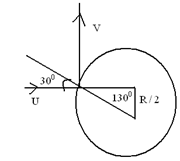Coefficient of restitution
Question

# A particle moving horizontally with a velocity u strikes a fixed frictionless sphere at a height R/2 above the center of sphere. After striking the sphere, velocity of particle changes to vertically upward .If R be the radius of sphere, the maximum height attained by the particle with respect to center of sphere is

Moderate
Solution

## Since the force of contact will be only normal force, $\therefore$The velocity of the particle along the tangent will be conserved during the collision$\begin{array}{l}\mathrm{Usin}30=\mathrm{Vsin}60\\ \mathrm{V}=\frac{\mathrm{U}}{\sqrt{3}}\end{array}$Maximum height from centre$=\frac{\mathrm{R}}{2}+\frac{{\mathrm{V}}^{2}}{2\mathrm{g}}=\frac{\mathrm{R}}{2}+\frac{{\mathrm{U}}^{2}}{6\mathrm{g}}$

Get Instant Solutions